# A new hard phase and physical properties of Tc2C predicted from first principles

Gangtai Zhanga, Yaru Zhaoa, Tingting Baib, Qun Weic and Yuquan Yuan*d
aCollege of Physics and Optoelectronics Technology, Baoji University of Arts and Sciences, Baoji 721016, PR China
bCollege of Mathematics and Information Science, Baoji University of Arts and Sciences, Baoji 721013, PR China
cSchool of Physics and Optoelectronic Engineering, Xidian University, Xi'an 710071, PR China
dSchool of Science, Sichuan University of Science and Engineering, Zigong 643000, PR China. E-mail: yuquan_yuan1975@suse.edu.cn

Received 6th May 2016 , Accepted 6th July 2016

First published on 6th July 2016

## Abstract

Using the first principles particle swarm optimization algorithm for crystal structural prediction, we have predicted a hexagonal P63/mmc structure of Tc2C. The new phase is mechanically and dynamically stable, as verified by its elastic constants and phonon dispersion. The formation enthalpy–pressure curves show that the predicted P63/mmc-Tc2C is more energetically favorable than the previously proposed Mo2C-, anti-MoS2-, Re2P-, and Fe2N-type structures in the considered pressure range. The calculated mechanical properties show that it is an ultra-incompressible and hard material. Meanwhile, the directional dependences of the Young's modulus, bulk modulus, and shear modulus for Tc2C are systematically investigated. The analyses of the density of states and electronic localization function reveal the presence of strong covalent bonding between Tc and C atoms, which is of crucial importance in forming a hard material.

## 1. Introduction

Designing and searching for ultra-incompressible and superhard materials are of great scientific interest due to their various industrial applications, such as cutting and polishing tools, abrasives, oil exploitations, and coatings. Previously, it was generally accepted that superhard materials are strong covalently bonded compounds formed by light atoms B, C, N, and O, such as diamond,1 c-BN,2 B6O,3 etc. These materials easily form strong three-dimensional covalent bonding networks. Though diamond is the hardest known material with a measured hardness of 60–120 GPa, it is unstable in the presence of oxygen at a moderate temperature and reacts easily with iron-based materials. The second-hardest material is cubic boron nitride (c-BN), but it can be synthesized only under high temperature and high temperature conditions, which results in a great cost of synthesis. Thus, intense effort has been devoted for hunting the new hard and ultra-incompressible materials. Recently, it has been reported that the incorporation of light atoms (B, C, N and O) into heavy transition metals (TMs) with high valence electron densities provides a good candidate for designing new hard materials. The compounds formed by transition metal and light atoms usually possess high valence electron density and directional covalent bonds, and these covalent bonds are strong enough to inhibit creation and movement of dislocations, which significantly improve their mechanical properties and create high hardness. Following this design criterion, recent design of the new intrinsically potential superhard materials has focused on light element TM compounds, e.g. ReB2,4 WC,5 IrN2,6 RuO27 and so on, the obtained results show that these materials usually possess the large bulk and shear moduli. Thereby, these pioneering studies open up a new route for pursuing new superhard materials.

Carbides, especially TM carbides, are widely used for industrial application because of their high melting temperature, extreme hardness, and chemical stability, which makes them useful in cutting tools, dental drills, rock drills in mining, and abrasives. Experimentally, some monocarbides, such as TiC,8 ZrC,9 PtC,10 and WC5 have been synthesized, the obtained results show that they all hold very bulk modulus. A high bulk modulus is a good indicator of a superhard material, thus TM carbides are potential candidates for superhard materials. Re2C belongs to the family of TM carbides, and some experimental and theoretical studies of Re2C have been investigated.11–15 Meanwhile, these theoretical calculations and experimental data reveal that Re2C is ultra-incompressible and has a high bulk module of about 400 GPa.

Technetium (Tc) lies directly above Re in the periodic table and has the same valence electron number, so it is worth studying the mechanical properties of its carbides. Experimentally, TcC has been first synthesized in 1962 and was presumed to be hexagonal and faced-centred cubic structures.16 Later, Giorgi and Szklarz interpreted the cubic compound on the authority of a body-centred cubic phase.17 Recently, an experimental synthesis has verified the existence of TcC,18 which also provides a useful information for understanding the crystal structure of TcC. Theoretically, the crystal structures and the related physical properties of TcC have been considerably investigated.19–25 These calculated results indicated that TcC with considered structures is an ultra-incompressible and hard material. However, the research works of the compounds with lower carbon contents are seldom reported so far.

In this paper, we have extensively investigated the ground state structure of Tc2C by using the first principles particle swarm optimization algorithm (PSO) on crystal structural prediction.26 This method has been successfully applied to various systems,27–29 unbiased by any known information. A novel hexagonal P63/mmc structure is uncovered for Tc2C, which is more energetically favorable than the earlier proposed Mo2C-, anti-MoS2-, Re2P-, and Fe2N-type structures in the pressure range of 0–120 GPa. First principles calculations are then performed to study the total energy, lattice parameters, phase stability, elastic properties, elastic anisotropy, and density of states for this novel hexagonal phase.

## 2. Computational methods

The PSO technique for crystal structural prediction has been implemented in the crystal structure analysis by the particle swarm optimization (CALYPSO) code30 at 0 GPa with 1–4 formula units (f.u.) each simulation cell. The underlying ab initio calculations are performed using density functional theory as implemented in the Vienna ab initio simulation package (VASP).31 The generalized gradient approximation (GGA) is used for the exchange and correlation potentials. The all-electron projector augmented wave (PAW) method32 is employed with 2s22p2 and 4p64d55s2 treated as the valence electrons for C and Tc, respectively. Geometry optimization is performed by using the conjugate gradient algorithm method with a plane-wave cutoff energy of 520 eV. The calculations are conducted with 14 × 14 × 4, 14 × 14 × 4, 8 × 6 × 7, 14 × 14 × 4, 7 × 12 × 4, and 14 × 14 × 4 for the predicted P63/mmc-Tc2C structure and our considered anti-ReB2-type (space group P63/mmc), Mo2C-type (space group Pbcn), anti-MoS2-type (space group P63/mmc), Re2P-type (space group Pnma), and Fe2N-type (space group P-3m1) structures, respectively. For hexagonal structures, Γ centered k mesh is adopted, and for other structures, Monkhorst–Pack k meshes33 are used to ensure that all the total energy calculations are well converged to better than 1 meV per atom. The phonon calculation is carried out by using a supercell approach as implemented in the PHONOPY code.34 Single crystal elastic constants are calculated by a strain-energy approach, i.e., applying a small strain to the equilibrium lattice and fitting the dependence of the resulting change in energy on the strain. The bulk modulus, shear modulus, Young's modulus, and Poisson's ratio are derived from the Voigt–Reuss–Hill approximation.35

## 3. Results and discussion

Using the PSO technique, we perform variable-cell structure prediction simulation for Tc2C containing 1–4 f.u. in the simulation cell at 0 GPa, a hexagonal P63/mmc structure is found, as shown in Fig. 1. The P63/mmc structure contains two Tc2C f.u. in a unit cell. At ambient pressure, the equilibrium lattice parameters are a = b = 2.838 Å and c = 9.774 Å, in which the Tc and C atoms occupy Wyckoff 4f (1/3, 2/3, 0.89092) and 2c (1/3, 2/3, 1/4) sites, respectively. From Fig. 1(a), each C atom is coordinated by 6 neighboring Tc atoms, forming edge-shared Tc6C trigonal prisms with Tc–C bond distance of 2.141 Å, which is shorter than the Re–C (2.16 Å) and Os–C distance (2.32 Å) in hard materials Re2C11 and Os2C,36 respectively. To check the structural stability of the new phase of Tc2C, the phonon dispersion curves are calculated at 0 GPa and 120 GPa, respectively, and the results are given in Fig. 2. Clearly, the P63/mmc structure is dynamically stable at ambient pressure and high pressure because of the inexistence of the imaginary phonon frequency in the whole Brillouin zone. It is well known that the thermodynamic stability of a compound can be described by the knowledge of its ground-state energy. Therefore, we calculate the total energy per f.u. as a function of the volume for the predicted P63/mmc structure. For comparison, the previously known five structures of anti-ReB2, Mo2C, anti-MoS2, Re2P, and Fe2N are also considered for Tc2C. The calculated results are shown in Fig. 3. As seen from this figure, the predicted P63/mmc structure for Tc2C has the lower energy minimum than the Mo2C-, anti-MoS2-, Re2P-, and Fe2N-type structures but the same energy–volume curve as the anti-ReB2-type structure. On the one hand, this indicates that the predicted P63/mmc structure is the ground-state phase at 0 GPa. On the other hand, this confirms that the P63/mmc-Tc2C has the same structure with anti-ReB2.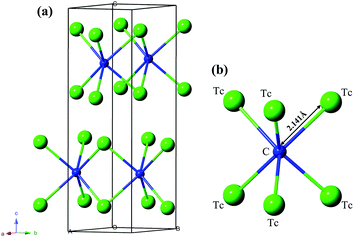Fig. 1 Crystal structure of the P63/mmc-Tc2C structure. Large and small spheres represent Tc and C atoms, respectively.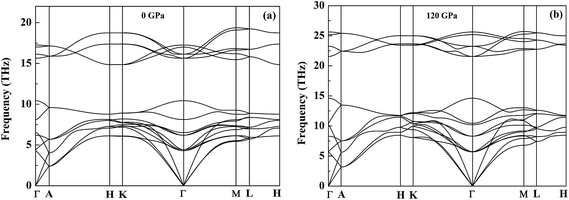Fig. 2 Phonon dispersion curves of the P63/mmc-Tc2C at 0 GPa (a) and 120 GPa (b).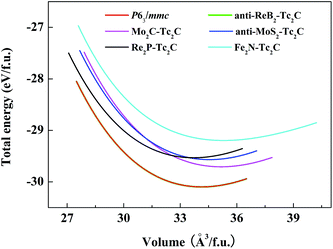Fig. 3 Total energy versus f.u. volume for Tc2C with five different structures.

To explore the thermodynamic stability for further experimental synthesis, the formation enthalpy of the Tc2C with respect to the separate phases is examined by the reaction route ΔH = HTc2C − 2HTcHc, here ΔH is the formation enthalpy, the hexagonal Tc (space group: P63/mmc) and the graphite C are chosen as the reference phases. The calculated formation enthalpy under pressure is shown in Fig. 4. As shown in this figure, the stabilities of Tc2C with different structures are gradually enhanced with increasing the pressure, therefore, the pressure is helpful to their stabilities. At ambient condition, the negative values of the formation enthalpies for the predicted P63/mmc-Tc2C and anti-ReB2-type structures indicate that they are thermodynamically stable, whereas the positive formation enthalpies for other structures show that they are not thermodynamically stable. Moreover, it is can be seen that the enthalpy–pressure curves of the P63/mmc- and anti-ReB2-type structures merge together in our considered pressure range, which further proves that they belong to the same structure. The present calculated results suggest that the predicted P63/mmc-Tc2C can be synthesized at ambient condition, thus further experimental synthesis is highly desirable.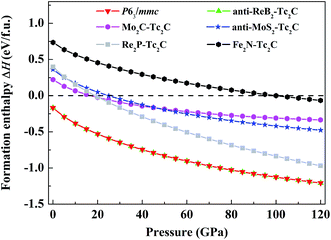Fig. 4 The calculated formation enthalpy–pressure curves for Tc2C with five different structures.

As is well known, the mechanical stability is a necessary condition for the stabilization of a crystal to exist, and the mechanical properties, such as elastic constants and elastic moduli, are important for potential technological and industrial applications. Thus we perform further the studies on the mechanical properties of the P63/mmc-Tc2C phase. By using a strain-energy method, we obtain the zero-pressure elastic constants (Cij) of the P63/mmc-Tc2C and P-6m2-TcC, respectively. The calculated elastic constants Cij are listed in Table 1, along with the theoretical values and available experimental data of other TM carbides (WC,5 PtC,10 Re2C,11,12,14,15 Ru2C,37,38 and Os2C36,39) for comparison. For a stable hexagonal crystal, Cij should satisfy the following criteria:40 C33 > 0, C44 > 0, C12 > 0, C11 > |C12|, (C11 + 2C12)C33 > 2C132. As shown in Table 1, the elastic constants of the predicted P63/mmc-Tc2C satisfy completely the elastic stability criteria for a hexagonal crystal, thus it is mechanically stable at ambient condition. The large values of C11 and C33 for the compound suggest that it is extremely difficult to be compressed along a-axis (or b-axis) and c-axis, respectively. Moreover, the C33 value of the P63/mmc phase (781 GPa) is close to those for WC,5 TcC,19–21 and Re2C11 but much larger than Ru2C38 and Os2C,39 suggesting its high linear incompressibility along the c-axis. Based on the obtained elastic constants Cij, the polycrystalline bulk modulus B and shear modulus G are thus determined by the Voigt–Reuss–Hill approximation. The Young's modulus E and Poisson's ratio v can be derived by the following equations E = 9BG/(3B + G) and v = (3B − 2G)/(6B + 2G). The calculated bulk modulus, shear modulus, Young's modulus, and Poisson's ratio of the P63/mmc phase together with the reference materials mentioned above are listed in Table 1. As shown in Table 1, the bulk modulus of the P63/mmc phase is 343 GPa, which is comparable to the experimental data of WC (439 GPa),5 PtC (301(±15)),10 and Re2C (405(30) and 386(10))14,15 but much more higher than that of Ru2C (178(4) GPa),37 indicating its ultra-incompressible structure nature. Moreover, the calculated bulk modulus (B = 343 GPa) for the P63/mmc-Tc2C agrees well with that directly obtained from the fitting results (B0 = 344 GPa) of the third-order Birch–Murnaghan equation of states, further verifying the reliability of the present elastic calculations. In order to further compare the incompressibility of the P63/mmc-Tc2C, WC, PtC, Re2C, and Ru2C under pressure, the volume compressions as a function of the pressure are presented in Fig. 5. It can be seen that the volume incompressibility of the P63/mmc-Tc2C is comparable to those of WC and Re2C but exceeds those of PtC and Ru2C. Compared with the bulk modulus, the shear modulus of a material quantifies its resistance to the shear deformation and acts as a better indicator of the potential hardness. Obviously, the predicted P63/mmc phase has a large shear modulus of 216 GPa, thus it is expected to withstand the shear strain to a large extent. In addition, Young's modulus can also provide a good measure of the stiffness of materials except the bulk modulus and shear modulus. The larger Young's modulus the material has, the harder it is to deform. The calculated Young's modulus of the P63/mmc-Tc2C is 536 GPa, indicating that it is a hard material. Poisson's ratio v is a crucial parameter to describe the degree of directionality of the covalent bonding. Generally speaking, the typical v value is 0.1 for covalent materials and 0.33 for metal materials, respectively. From Table 1, it can be seen that the v value of the P63/mmc-Tc2C phase is below 0.33 and at the same time the P63/mmc-Tc2C has the smallest Poisson's ratio 0.239 in contrast to other considered TM carbides, such as Ru2C,38 Os2C,39 and TcC.19–21 Consequently, the P63/mmc-Tc2C has a strong degree of covalent boding. Furthermore, the B/G ratio is commonly used to predict the brittle or ductile behavior of materials. According to the Pugh criterion,41 if B/G > 1.75, the material behaves in a ductile way, otherwise, the material behaves in a brittle manner. For the case of the P63/mmc-Tc2C, our predicted value of B/G is 1.59, suggesting that it has a little brittle nature. Using the empirical formula for the hardness prediction proposed by Chen et al.,42 the estimated Vickers hardness for the P63/mmc-Tc2C is 24.11 GPa, which is comparable to the known hard materials of SiO2 (30.6 GPa)43 and B4C (32.8 GPa).44 All of these excellent mechanical properties strongly support that the P63/mmc-Tc2C is an ultra-incompressible and hard material.

Table 1 Calculated elastic constants Cij, bulk modulus B (GPa), EOS fitted bulk modulus B0 (GPa), shear modulus G (GPa), Young's modulus E (GPa), B/G, and Poisson's ratio v, Debye temperature Θ (K) for P63/mmc-Tc2C and P-6m2-TcC, together with other theoretical results and available experimental data
Structure   C11 C12 C13 C33 C44 C66 B B0 G E B/G v Θ
a Our calculated result from ref. 39 on the basis of v = (3B − 2G)/(6B + 2G).
P63/mmc-Tc2C This work (GGA) 612 201 172 781 199 206 343 344 216 536 1.59 0.239 675
P63/mmc-Re2C Theor. (GGA)11 748 201 219 939 252 273 410 400 273 671   0.227 564
Theor.12             388.9
Expt.14             405(30)
Expt.15             386(10)
P-3m1-Ru2C Expt.37             178(4)
P-31m-Ru2C Theor. (GGA)38 500 198 239 481 151   315   139   2.27 0.307a
P63/mmc-Os2C Theor. (GGA)36               418.48
Theor. (GGA)39 639 142 329 579 111   381   152 402 2.51 0.32
P-6m2-TcC This work (GGA) 682 200 166 940 169 241 371 372 225 562 1.65 0.247 756
Theor. (LDA)19 749 244 211 1031 199 252 426   270   1.59 0.24 831
Theor. (LDA)20 752 228 199 1030 198     416 252 629   0.249
Theor. (LDA)21 759 232 188 1044 188   414 416 249 623 1.67 0.249 864
P-6m2-WC Expt.5 720 254 267 972 328   439
Fm-3m-PtC Expt.10             301(±15)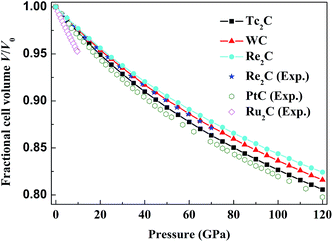Fig. 5 The calculated volume compression as a function of pressure for the P63/mmc-Tc2C compared with WC, PtC, Re2C, and Ru2C.

For engineering applications that make use of single crystals, it is necessary to know the values of the Young's modulus, bulk modulus, and shear modulus as a function of crystal orientation. For the hexagonal P63/mmc-Tc2C, the Young's modulus and bulk modulus are expressed by:

 E−1 = s11(α2 + β2)2 + s33γ4 + (2s13 + s44)(β2γ2 + α2γ2), (1)
 B−1 = (s11 + s12 + s13) − (s11 + s12 − s13 − s33)γ2, (2)
where α, β, and γ are the direction cosines of [uvw] direction and sij are the elastic compliance constants which are obtained by Nye.45 The shear modulus on the (hkl) shear plane with the shear stress applied alone the [uvw] direction is written as
 G−1 = 4s11(α12α22 + β12β22) + 4s33γ12γ22 + 8s12α1α2β1β2 + 8s13(α1α2 + β1β2)γ1γ2 + s44[(β1γ2 + β2γ1)2 + (α1γ2 + α2γ1)2] + s66(α1β2 + α2β1)2, (3)
where α1, β1, γ1, α2, β2, γ2 are the direction cosines of the [uvw] and [hkl] directions in the coordinate systems, and the [hkl] direction shows the vector normal to the (hkl) shear plane.

Fig. 6(a) and (c) are the three-dimensional surface representations which show the variation of the Young's modulus and bulk modulus, respectively. For an isotropic crystal, one would see a spherical shape, while a deviation from a spherical shape can directly reflect the degree of the elastic anisotropy in the crystal. From these two figures, one can see that the Young's modulus exhibits a high degree of the elastic anisotropy due to a large deviation from a spherical shape, whereas the bulk modulus is nearly isotropic because of a small deviation from a spherical shape. The projections of the Young's modulus and bulk modulus on the ab and bc planes are also plotted in Fig. 6(b) and (d) for comparison. As can be seen, in-plane anisotropy in the ab plane is not existent, whereas in-plane anisotropy in the bc plane is directly revealed. A deeper insight of the change of the Young's modulus alone different directions can be obtained by investigating the directional dependences of the Young's modulus alone tensile axis in the (0001), (100), (1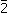12), and (012) planes, respectively, and the obtained results are given in Fig. 7(a). For the (0001) plane, since the direction cosines are α = cosθ, β = sinθ, and γ = 0 (θ is the angle between tensile stress and ), one obtains E−1 = s11 from eqn (1). This implies that the Young's modulus on the basal plane is independent of the tensile stress direction for the P63/mmc-Tc2C, which is the reason of the elastic isotropy in the basal plane for hexagonal crystal. For the directional dependences of the Young's modulus from  to [20] in the (100) plane, the P63/mmc-Tc2C possesses a maximum of E = 708.2 GPa and a minimum of E[20] = 527.8 GPa. For the (112) plane, the Young's modulus alone the [100] direction has the minimal value (Emin = 527.8 GPa) and the Young's modulus alone the [21] direction has the maximal one (Emax = 653.6 GPa). For the change of the Young's modulus in the (012) plane for the quadrant of directions between [20] and [101], the P63/mmc-Tc2C exhibits a maximum of E[101] = 591.7 GPa and a minimum of E[20] = 527.8 GPa. Consequently, the order of the Young's modulus as a function of the principal crystal tensile [uvw] for the P63/mmc-Tc2C is: E > E[21] > E[101] > E[100]. To understand the plastic deformation for the predicted P63/mmc-Tc2C phase, we investigate the dependence of the shear modulus on the stress direction, and the corresponding result is presented in Fig. 7(b). For the (0001) shear plane with the shear stress direction varied from [100] to [20], since the direction cosines are α1 = β1 = 0, γ1 = 0, α2 = cosθ, β2 = sinθ, and γ2 = 0 (θ is the angle between the shear direction and [100]), one can obtain G−1 = s44 from eqn (3). This implies that the shear modulus is isotropic in the basal plane, as shown by the green curve in Fig. 7(b). For the shear plane (100) with the shear stress direction changed from  to [20], α1 = 1, β1 = γ1 = 0, α2 = 0, β2 = sinθ, and γ2 = cosθ (θ is the angle between the shear direction and ), so the shear module can be reduced to G−1 = s66 + (s44s66)cos2θ. Since s44 > s66 in our calculations, the shear module on the (100) plane is the highest when θ = 90° and the lowest when θ = 0, corresponding to Gmax = 205.5 GPa for the [20] direction and Gmin = 199 GPa for the  direction. For the shear plane (112) with the shear stress direction rotated from [100] to [21], the maximum of the shear modulus is 212.6 GPa for the [21] direction and the minimum of the shear modulus is 204.9 GPa for the [100] direction. When the pyramidal plane (012) is the shear plane and the shear stress direction is changed from [20] to [101], the shear modulus is the largest alone the [101] direction (Gmax = 232.4 GPa) and the shear modulus is the smallest along the [20] direction (Gmin = 204.2 GPa). Through the above analysis, we draw conclusions: (1) the Young's modulus and shear modulus are constant on the basal plane and orientation-dependent on both the pyramidal and prismatic planes; (2) the bulk modulus is almost isotropic.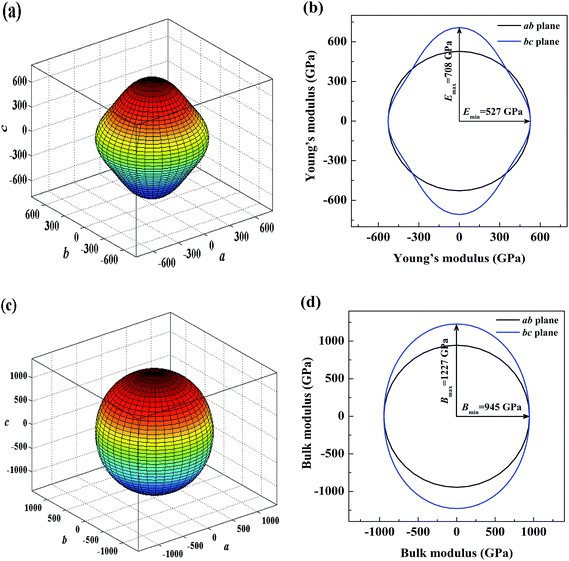Fig. 6 Three-dimension surface representations of the Young's modulus (a) and bulk modulus (c) in the P63/mmc-Tc2C. The plane projections of the directional dependence of the Young's modulus (b) and bulk modulus (d) in the P63/mmc-Tc2C.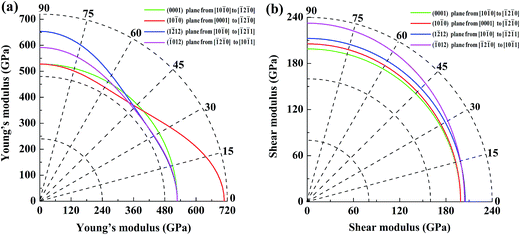Fig. 7 Directional dependences of Young's modulus (a) and the shear modulus (b) in the (0001), (100), (012), and (112) planes.

The elastic anisotropy of crystals can exert great effects on the properties of the physical mechanism, such as anisotropic plastic deformation, crack behavior, and elastic instability. Therefore, it is important and necessary to investigate the elastic anisotropy to improve their mechanical durability. The compression and the shear anisotropic factors provide the measures of the degrees of anisotropy in atomic bonding in different crystallographic planes. For hexagonal crystal, the anisotropies in compressibility and shear are defined as:

 (4)
 (5)

For an isotropic crystal, the factors Acomp and Ashear must be one, while any departure from one is a measure of the degree of elastic anisotropy possessed by the crystal. According to the above definitions, the values of Acomp and Ashear are calculated to be 0.77 and 0.968, respectively. This indicates that the P63/mmc-Tc2C phase has a certain degree elastic anisotropy.

Debye temperature is related to many physical properties of materials, such as specific heat, elastic constants, and melting temperature.46 It is used to differentiate between high and low temperature regions for a solid. When the temperature T > ΘD, all modes are expected to have the energy of kBT; when T < ΘD, the high-frequency modes are expected to be frozen, namely the vibrational excitations origin only from the acoustic vibrations. The Debye temperature ΘD for the studied P63/mmc-Tc2C is estimated from the average sound velocity (vm) by the following equation:

 (6)
where h is Planck's constant, k is Boltzmann's constant, NA is Avogadro's number, n is the number of atoms per formula unit, M is the molecular mass per f.u., and ρ is the density. The average sound velocity vm is given by:
 (7)
where vt and vl are the transverse and longitudinal elastic wave velocities of the polycrystalline material, which can be determined by Navier's equation.47 The obtained Debye temperatures of the P63/mmc-Tc2C under pressure are presented in Fig. 8. At zero pressure and zero temperature, the Debye temperature for the predicted P63/mmc-Tc2C phase is 675 K. Generally, the higher Debye temperature the materials possess, the larger microhardness they have. From Fig. 8, one can see clearly that the Debye temperature increases with the increase of the pressure. This means that the pressure is better for the enhancement of the hardness for the P63/mmc-Tc2C.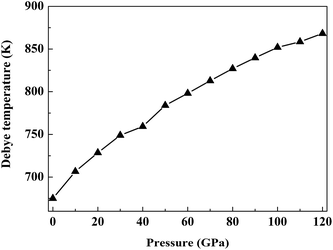Fig. 8 Debye temperature of the P63/mmc-Tc2C as a function of pressure.

The electronic structure is the key to understand the mechanical properties of the P63/mmc-Tc2C, the total and partial densities of states (DOS) are calculated and given in Fig. 9, where the vertical dashed line denotes the Fermi level. Distinctly, the P63/mmc-Tc2C exhibits a good metallic behavior due to the adequately large total DOS at the Fermi level. This metallicity might make it a better candidate for hard conductors. From partial DOS, it can be clearly seen that the peaks from −13 eV to −10.8 eV are mainly contributed by C-2s and Tc-4d states with small contributions from the 4p and 5s electrons of Tc. The states from −7.3 eV to the Fermi energy (0 eV) mainly originate from the Tc-4d and C-2p orbitals with small contributions of Tc-4p and Tc-5s. In addition, the partial DOS profiles of Tc-4d and C-2p are very similar in the energy region from −7.3 eV to 0 eV, which reflects that Tc-4d orbital has a significant hybridization with C-2p orbital. This fact also shows the existence of a strong covalent bonding between the Tc and C atoms. For the total DOS, the typical feature is the presence of so-called pseudogap, which is regarded as the borderline between the bonding states and antibonding states. Since the Fermi energy is located below the pseudogap for the P63/mmc-Tc2C phase, its bonding states are partially occupied and full antibonding states are unoccupied. This property also increases the structural stability of Tc2C. Fig. 10 presents the calculated electronic localization function (ELF) of the predicted P63/mmc-Tc2C phase on the (110) plane. From this figure, one can see that the large ELF value between the Tc and C atoms indicates the partially Tc–C covalent bonding interaction in the P63/mmc-Tc2C phase. This also explains the reason of the high bulk modulus and large shear modulus of Tc2C.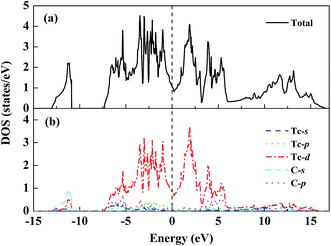Fig. 9 Total (a) and partial (b) densities of states of the P63/mmc-Tc2C. The vertical dashed line denotes the Fermi level EF.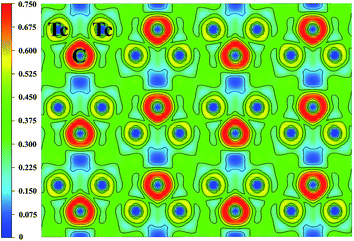Fig. 10 Contours of ELF of the P63/mmc-Tc2C on the (110) plane at 0 GPa.

## 4. Conclusions

In conclusion, a new hexagonal P63/mmc structure is unraveled to be the ground-state structure for Tc2C using the PSO algorithm, and it is energetically much superior to the previously known Mo2C-, anti-MoS2-, Re2P-, and Fe2N-type structures in the considered pressure range. Based on the calculated phonon dispersion and formation enthalpy curves, we demonstrate that the predicted P63/mmc phase is dynamically stable and synthesizable under the ambient condition. The high bulk modulus, large shear modulus, small Poisson's ratio, and considerable hardness indicate that the P63/mmc-Tc2C is an ultra-incompressible and hard material. Moreover, the Young's modulus, bulk modulus, and shear modulus as a function of crystal orientation for Tc2C are also investigated systematically. The presence of strong covalent Tc–C bonds, which is of crucial importance in forming a hard material, is confirmed by the detailed analyses of the electronic structure and electronic localization function. We expect that our theoretical results will stimulate further experimental research on this material in the future.

## Acknowledgements

This work was supported by the Science Foundation of Baoji University of Arts and Sciences of China (Grant No. ZK16069 and ZK11135), the Natural Science Foundation of the Education Committee of Shaanxi Province of China (Grant No. 2013JK0637), the Natural Science Basic Research plan in Shaanxi Province of China (Grant No. 2016JM1012), and the Natural Science Foundation of China (Grant No. 11404008).

## References

1. F. Occelli, D. L. Farber and R. L. Toullec, Nat. Mater., 2003, 2, 151 CrossRef CAS PubMed.
2. Y. Zhang, H. Sun and C. F. Chen, Phys. Rev. B: Condens. Matter Mater. Phys., 2006, 73, 144115 CrossRef.
3. D. W. He, Y. S. Zhao, L. Daemen, J. Qian, T. D. Shen and T. W. Zerda, Appl. Phys. Lett., 2002, 81, 643 CrossRef CAS.
4. H. Y. Chung, M. B. Weinberger, J. B. Levine, A. Kavner, J. M. Yang, S. H. Tolbert and R. B. Kaner, Science, 2007, 316, 436 CrossRef CAS PubMed.
5. M. Lee and R. S. Gilmore, J. Mater. Sci., 1982, 17, 2657 CrossRef CAS.
6. J. C. Crowhurst, A. F. Goncharov, B. Sadigh, C. L. Evans, P. G. Morrall, J. L. Ferreira and A. J. Nelson, Science, 2006, 311, 1275 CrossRef CAS PubMed.
7. J. S. Tse, D. D. Klug, K. Uehara, Z. Q. Li, J. Haines and J. M. Léger, Phys. Rev. B: Condens. Matter Mater. Phys., 2000, 61, 10029 CrossRef CAS.
8. N. A. Dubrovinskaia, L. S. Dubrovinsky, S. K. Saxena, R. Ahuja and B. Johansson, J. Alloys Compd., 1999, 289, 24 CrossRef CAS.
9. R. Chang and L. J. Graham, J. Appl. Phys., 1966, 37, 3778 CrossRef CAS.
10. S. Ono, T. Kikegawa and Y. Ohishi, Solid State Commun., 2005, 133, 55 CrossRef CAS.
11. H. Ozisik, E. Deligoz, K. Colakoglu and G. Surucu, Phys. Status Solidi RRL, 2010, 4, 347 CrossRef CAS.
12. Z. S. Zhao, L. Cui, L. M. Wang, B. Xu, Z. Y. Liu, D. L. Yu, J. L. He, X. F. Zhou, H. T. Wang and Y. J. Tian, Cryst. Growth Des., 2010, 10, 5025 Search PubMed.
13. E. A. Juarez-Arellano, B. Winkler, A. Friedrich, D. J. Wilson, M. Koch-Müller, K. Knorr, S. C. Voge, J. J. Wall, H. Reiche, W. Crichton, M. Ortega-Aviles and M. Avalos-Borja, Z. Kristallogr., 2008, 223, 492 CAS.
14. E. A. Juarez-Arellano, B. Winkler, A. Friedrich, L. Bayarjargal, V. Milman, J. Y. Yan and S. M. Clark, J. Alloys Compd., 2009, 481, 577 CrossRef CAS.
15. N. Yasui, M. Sougawa, M. Hirai, K. Yamamoto, T. Okada, D. Yamazaki, Y. Kojima, H. Ohfuji, S. Kunitsugu and K. Takarabe, Cogent Physics, 2015, 2, 1076702 CrossRef.
16. W. Trzebiatowski and J. Z. Rudzinski, Z. Chem., 1962, 2, 158 CrossRef CAS.
17. A. L. Giorgi and E. G. Szklarz, J. Less-Common Met., 1966, 11, 455 CrossRef CAS.
18. V. F. Peretrukhin, K. N. Gedgovd, M. S. Grigoriev, A. V. Tarasov, Y. V. Plekhanov, A. G. Maslennikov, G. S. Bulatov, V. P. Tarasov and M. Lecomteb, J. Nucl. Radiochem. Sci., 2005, 6, 211 CrossRef.
19. Y. X. Wang, Phys. Status Solidi RRL, 2008, 2, 126 CrossRef CAS.
20. Y. C. Liang, C. Li, W. L. Guo and W. Q. Zhang, Phys. Rev. B: Condens. Matter Mater. Phys., 2009, 79, 024111 CrossRef.
21. Y. C. Zou, J. Zhu, Y. J. Hao, G. Xiang, X. C. Liang and J. R. Wang, Phys. Status Solidi B, 2014, 251, 1372 CrossRef CAS.
22. X. W. Sun, Y. D. Chu, Z. J. Liu, T. Song, J. H. Tian and X. P. Wen, Chem. Phys. Lett., 2014, 614, 167 CrossRef CAS.
23. T. Song, Q. Ma, J. H. Tian, X. B. Liu, Y. H. Ouyang, C. L. Zhang and W. F. Su, Mater. Res. Bull., 2015, 61, 58 CrossRef CAS.
24. M. Kavitha, G. S. Priyanga, R. Rajeswarapalanichamy and K. Iyakutti, Int. J. Refract. Met. Hard Mater., 2015, 52, 219 CrossRef CAS.
25. Q. G. Wang, K. E. German, A. R. Oganov, H. F. Dong, O. D. Feya, Y. V. Zubavichus and V. Y. Murzin, RSC Adv., 2016, 6, 16197 RSC.
26. Y. C. Wang, J. Lv, L. Zhu and Y. M. Ma, Phys. Rev. B: Condens. Matter Mater. Phys., 2010, 82, 094116 CrossRef.
27. J. Lv, Y. C. Wang, L. Zhu and Y. M. Ma, Phys. Rev. Lett., 2011, 106, 015503 CrossRef PubMed.
28. L. Zhu, H. Wang, Y. C. Wang, J. Lv, Y. M. Ma, Q. L. Cui, Y. M. Ma and G. T. Zou, Phys. Rev. Lett., 2011, 106, 14550 Search PubMed.
29. Z. S. Zhao, B. Xu, L. M. Wang, X. F. Zhou, J. L. He, Z. Y. Liu, H. T. Wang and Y. J. Tian, ACS Nano, 2011, 5, 7226 CrossRef CAS PubMed.
30. Y. M. Ma, Y. C. Wang, J. Lv and L. Zhu, http://nlshm-lab.jlu.edu.cn/%7Ecalypso.html.
31. G. Kresse and D. Joubert, Phys. Rev. B: Condens. Matter Mater. Phys., 1999, 59, 1758 CrossRef CAS.
32. P. E. Blöchl, Phys. Rev. B: Condens. Matter Mater. Phys., 1994, 50, 17953 CrossRef.
33. H. J. Monkhorst and J. D. Pack, Phys. Rev. B: Solid State, 1976, 13, 5188 CrossRef.
34. A. Togo, F. Oba and I. Tanaka, Phys. Rev. B: Condens. Matter Mater. Phys., 2008, 78, 134106 CrossRef.
35. R. Hill, Proc. Phys. Soc., London, Sect. A, 1952, 65, 349 CrossRef.
36. N. R. Sanjay Kumar, S. Chandra, S. Amirthapandian, N. V. Chandra Shekar and P. C. Sahu, Mater. Res. Express, 2015, 2, 016503 CrossRef.
37. N. R. Sanjay Kumar, N. V. Chandra Shekar, S. Chandra, J. Basu, R. Divakar and P. Ch Sahu, J. Phys.: Condens. Matter, 2012, 24, 362202 CrossRef CAS PubMed.
38. J. Lu, F. Hong, W. J. Lin, W. Ren, Y. W. Li and Y. F. Yan, J. Phys.: Condens. Matter, 2015, 27, 175505 CrossRef PubMed.
39. L. P. Ding, P. Shao, F. H. Zhang, X. F. Huang and T. L. Yuan, J. Phys. Chem. C, 2015, 119, 21639 CAS.
40. M. Born, Proc. Cambridge Philos. Soc., 1940, 36, 160 CrossRef CAS.
41. S. F. Pugh, Philos. Mag., 1954, 45, 823 CrossRef CAS.
42. X. Q. Chen, H. Y. Niu, D. Z. Li and Y. Y. Li, Intermetallics, 2011, 19, 1275 CrossRef CAS.
43. F. M. Gao, J. L. He, E. D. Wu, S. M. Liu, D. L. Yu, D. C. Li, S. Y. Zhang and Y. J. Tian, Phys. Rev. Lett., 2003, 91, 015502 CrossRef PubMed.
44. G. Kresse and D. Joubert, Phys. Rev. B: Condens. Matter Mater. Phys., 1999, 59, 1758 CrossRef CAS.
45. J. F. Nye, Physical properties of Crystals: Their Representation by Tensors and Matrices, Oxford University Press, New York, 1985 Search PubMed.
46. P. Ravindran, L. Fast, P. A. Korzhavyi, B. Johansson, J. Wills and O. Eriksson, J. Appl. Phys., 1998, 84, 4891 CrossRef CAS.
47. R. Ahmed, F. E. Aleem, S. J. Hashemifar and H. Akbarzadeh, Phys. B, 2007, 400, 297 CrossRef CAS.
 This journal is © The Royal Society of Chemistry 2016